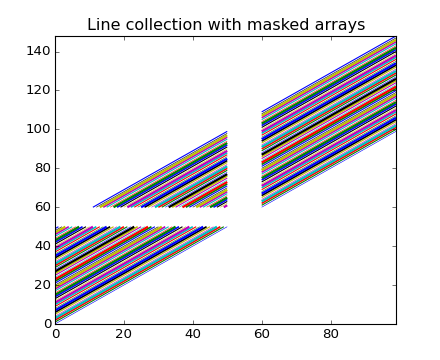#### Previous topic

pylab_examples example code: legend_demo4.py

#### Next topic

pylab_examples example code: line_collection2.py

# pylab_examples example code: line_collection.py¶```import matplotlib.pyplot as plt
from matplotlib.collections import LineCollection
from matplotlib.colors import colorConverter

import numpy as np

# In order to efficiently plot many lines in a single set of axes,
# Matplotlib has the ability to add the lines all at once. Here is a
# simple example showing how it is done.

x = np.arange(100)
# Here are many sets of y to plot vs x
ys = x[:50, np.newaxis] + x[np.newaxis, :]

segs = np.zeros((50, 100, 2), float)
segs[:,:,1] = ys
segs[:,:,0] = x

segs = np.ma.masked_where((segs > 50) & (segs < 60), segs)

# We need to set the plot limits.
ax = plt.axes()
ax.set_xlim(x.min(), x.max())
ax.set_ylim(ys.min(), ys.max())

# colors is sequence of rgba tuples
# linestyle is a string or dash tuple. Legal string values are
#          solid|dashed|dashdot|dotted.  The dash tuple is (offset, onoffseq)
#          where onoffseq is an even length tuple of on and off ink in points.
#          If linestyle is omitted, 'solid' is used